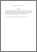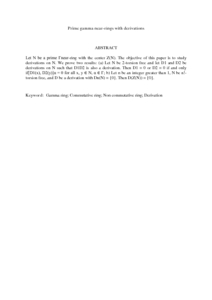# Prime gamma near-rings with derivations

## Citation

Rakhimov, Isamiddin Sattarovich and Dey, Kalyan Kumar and Paul, Akhil Chandra (2013) Prime gamma near-rings with derivations. International Journal of Pure and Applied Mathematics, 83 (2). pp. 221-231. ISSN 1311-8080; ESSN: 1314-3395

## Abstract

Let N be a prime Γnear-ring with the center Z(N). The objective of this paper is to study derivations on N. We prove two results: (a) Let N be 2-torsion free and let D1 and D2 be derivations on N such that D1D2 is also a derivation. Then D1 = 0 or D2 = 0 if and only if[D1(x), D2(y)]α = 0 for all x, y ∈ N, α ∈ Γ; b) Let n be an integer greater than 1, N be n!-torsion free, and D be a derivation with Dn(N) = {0}. Then D(Z(N)) = {0}.Preview
PDF (Abstract)
Prime gamma near.pdfView Item